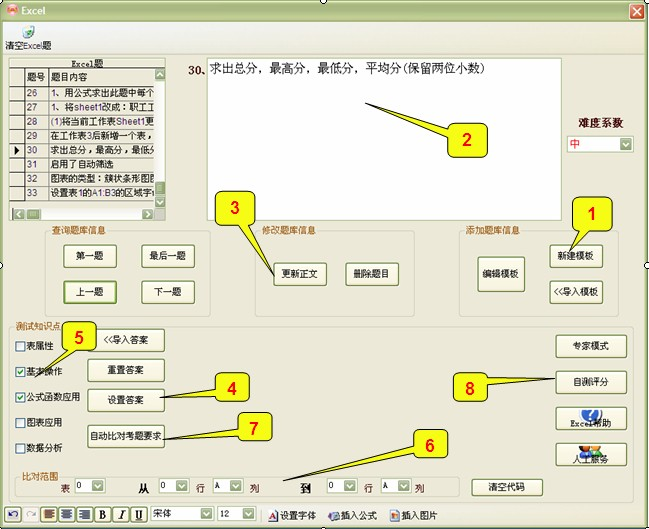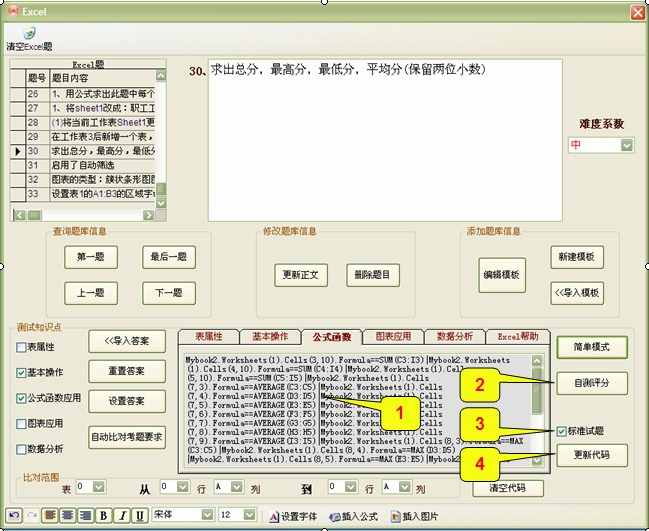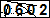1、新建模板

2、添加正文内容

3、更新正文

4、设置答案

5、选择测试知识点

6、选择比对的范围 (范围越小，比对时间越少)

7、自动比对考题要求(软件读取标准答案模板并转化为评分代码)

8、自测评分。 (功能：优化评分代码，做三次即可，每次需评得100分)

完毕！！Mybook2.Worksheets(1).Cells(3,10).Formula==SUM(C3:I3)|Mybook2.Worksheets(1).Cells(4,10).Formula==SUM(C4:I4)|Mybook2.Worksheets(1).Cells(5,10).Formula==SUM(C5:I5)|Mybook2.Worksheets(1).Cells(7,3).Formula==AVERAGE(C3:C5)|............

Mybook2.Worksheets(1).Cells(3,10).Formula==SUM(C3:I3)
'工作簿第一个工作表中的单元格（3，10）用到了公式函数计算数值C3至I3的数值总和

Mybook2.Worksheets(1).Cells(4,10).Formula==SUM(C4:I4)
'工作簿第一个工作表中的单元格（4，10）用到了公式函数计算数值C4至I4的数值总和

Mybook2.Worksheets(1).Cells(5,10).Formula==SUM(C5:I5)
'工作簿第一个工作表中的单元格（5，10）用到了公式函数计算数值C5至I5的数值总和

Mybook2.Worksheets(1).Cells(7,3).Formula==AVERAGE(C3:C5)
'工作簿第一个工作表中的单元格（7，3）用到了公式函数计算数值C3至C5的数值平均值

1、修改代码
2、

3、标准试题：设置为标准试题后，此题就不能被用户更改（建议检测代码评得100分的试题都设置成标准试题）

4、更新代码Excel帮助:
Mybook2.Worksheets(1).Cells(7,3).NumberFormat =0.00_ |保留两位小数|Mybook2.Worksheets(1).Cells(7,4).NumberFormat =0.00_ |保留两位小数|.........

Mybook2.Worksheets(1).Cells(7,3).NumberFormat =0.00_      对应   单元格（7,3） 格式类型 数值保留两位小数
Mybook2.Worksheets(1).Cells(7,4).NumberFormat =0.00_      对应   单元格（7,4） 格式类型 数值保留两位小数

1、添加解释
2、更新代码（保存代码）

• 发表评论
• 用户：  验证码：Keywords: 考试系统 考试系统